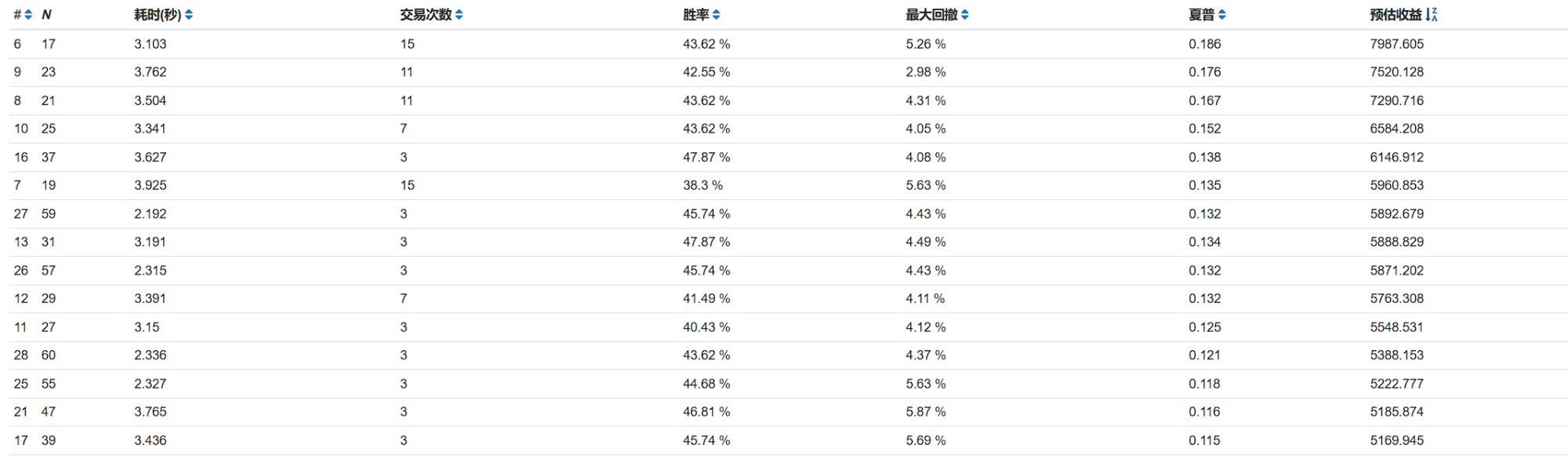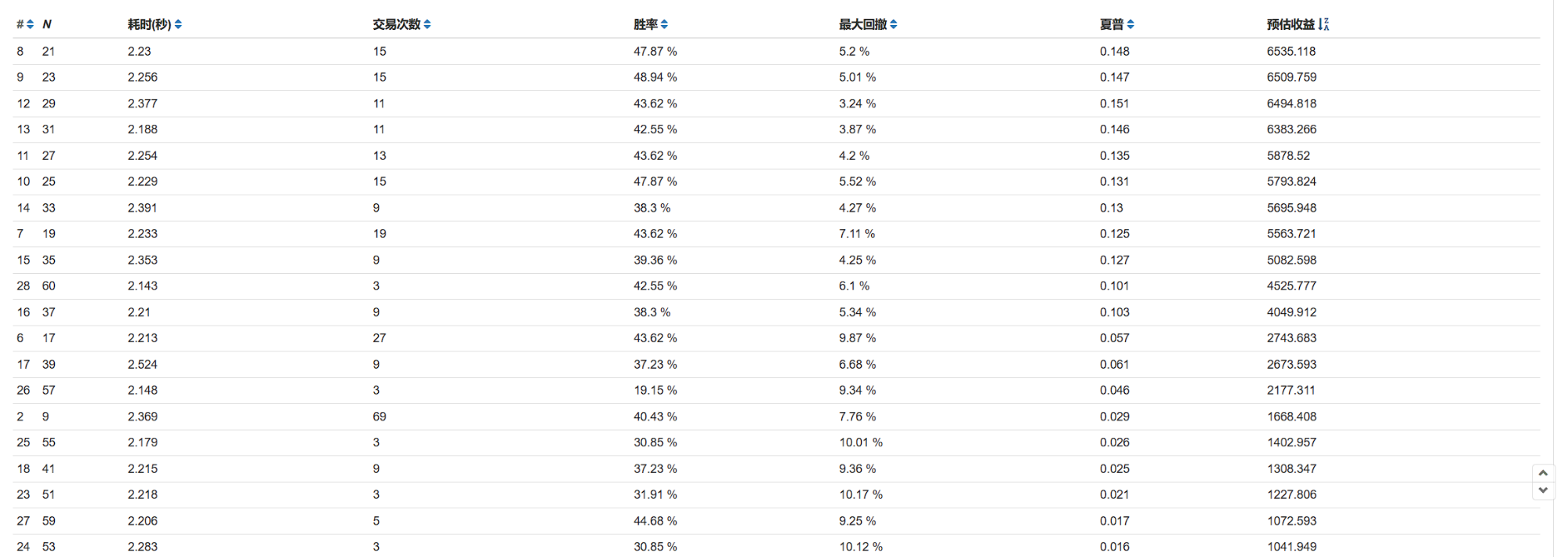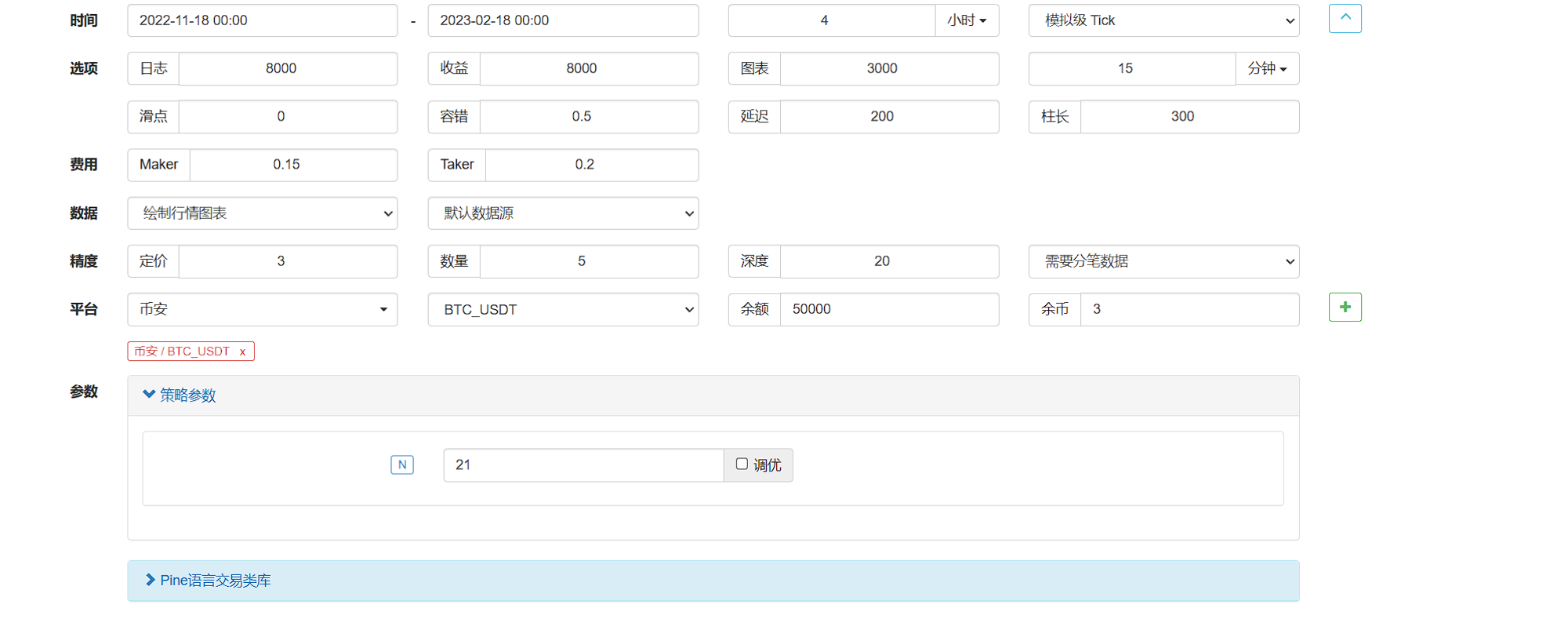ooFFiioo

V1

2023/02/19阅读：37主题：全栈蓝

# Q&A

## ADTM 中的 DTM 和 DBM 做了什么？

DTM 和 DBM 的值分别反映了当日市场买气和卖气的强度。在 ADTM 中，通过将 DTM 和 DBM 分别在一段时间内求和，再用两者之差除以二者之和的最大值，得到 ADTM 值，从而衡量市场人气的方向和强度。

## 为什么 STM 要将 20 天的 DTM 求和，乐观的情绪能够在 20 天中积累吗？

STM 和 SBM 分别是 20 天内市场的上涨动量和下跌动量的累计值。如果 STM 大于 SBM，说明市场买盘力量比卖盘力量强，市场情绪较为乐观，ADTM 值将大于 0。反之，如果 STM 小于 SBM，说明市场卖盘力量比买盘力量强，市场情绪较为悲观，ADTM 值将小于 0。如果 STM 等于 SBM，说明市场上涨和下跌的力量相当，市场情绪比较平静，ADTM 值将等于 0。

# 代码

``````//@version=5

n = input(20, title="N")

o = open

h = high

l = low

DTM = if o > o

math.max(h - o, o - o)

else

0

DBM = if o < o

math.max(o - l, o - o)

else

0

STM = math.sum(DTM, n)

SBM = math.sum(DBM, n)

ADTM = (STM - SBM) / math.max(STM, SBM)

plot(STM,color = color.blue)

plot(SBM,color = color.red)

// 绘制买入和卖出信号箭头

// plotshape(buySignal, title="Buy Signal", location=location.belowbar, style=shape.arrowup, color=color.green,size = size.auto,text = '买',textcolor = color.black)

// plotshape(sellSignal, title="Sell Signal", location=location.abovebar, style=shape.arrowdown, color=color.red,size = size.auto,text = '卖',textcolor = color.black)

strategy.entry("买入信号",strategy.long,1)

if (sellSignal)

strategy.entry("卖出信号",strategy.short,1)
``````

## Python 实现

``# ADTM 指标"""N=20DTM=IF(OPEN>REF(OPEN,1),MAX(HIGH-OPEN,OPEN-REF(OPEN,1)),0)DBM=IF(OPEN<REF(OPEN,1),MAX(OPEN-LOW,REF(OPEN,1)-OPEN),0)STM=SUM(DTM,N)SBM=SUM(DBM,N)ADTM=(STM-SBM)/MAX(STM,SBM)ADTM 通过比较开盘价往上涨的幅度和往下跌的幅度来衡量市场的人气。ADTM 的值在-1 到 1 之间。当 ADTM 上穿 0.5 时，说明市场人气较旺；当 ADTM 下穿-0.5 时，说明市场人气较低迷。我们据此构造交易信号。当 ADTM 上穿 0.5 时产生买入信号；当 ADTM 下穿-0.5 时产生卖出信号。"""df['h_o'] = df['high'] - df['open']df['diff_open'] = df['open'] - df['open'].shift(1)max_value1 = df[['h_o', 'diff_open']].max(axis=1)df.loc[df['open'] > df['open'].shift(1), 'DTM'] = max_value1df['DTM'].fillna(value=0, inplace=True)df['o_l'] = df['open'] - df['low']max_value2 = df[['o_l', 'diff_open']].max(axis=1)# DBM = pd.where(df['open'] < df['open'].shift(1), max_value2, 0)df.loc[df['open'] < df['open'].shift(1), 'DBM'] = max_value2df['DBM'].fillna(value=0, inplace=True)df['STM'] = df['DTM'].rolling(n).sum()df['SBM'] = df['DBM'].rolling(n).sum()max_value3 = df[['STM', 'SBM']].max(axis=1)df[factor_name] = (df['STM'] - df['SBM']) / max_value3``

# 一种可能的改进

1. 计算中间价（MM）= （最高价+最低价+2*收盘价）/4
2. 计算 CR（n 日）= n 日内上升总值之和 / n 日内下降总值之和 其中，上升总值为 n 日内（MM 比前一日高）的股票个数之和，下降总值为 n 日内（MM 比前一日低）的股票个数之和。
3. 计算 CRMA（m 日）= CR 的 m 日简单移动平均值 CR 指标的取值范围在 0-100 之间，一般认为当 CR 指标值高于 50 时，市场处于多头状态，当 CR 指标值低于 50 时，市场处于空头状态。

``````//@version=5

n = input(20, title="N")

o = open

h = high

l = low

c = close

average=(h+l+2*c)/4

DTM = if o > o

math.max(h - average, average - c)

else

0

DBM = if o < o

math.max(average - l, c - average)

else

0

STM = math.sum(DTM, n)

SBM = math.sum(DBM, n)

ADTM = (STM - SBM) / math.max(STM, SBM)

plot(STM,color = color.blue)

plot(SBM,color = color.red)

// 绘制买入和卖出信号箭头

// plotshape(buySignal, title="Buy Signal", location=location.belowbar, style=shape.arrowup, color=color.green,size = size.auto,text = '买',textcolor = color.black)

// plotshape(sellSignal, title="Sell Signal", location=location.abovebar, style=shape.arrowdown, color=color.red,size = size.auto,text = '卖',textcolor = color.black)

strategy.entry("买入信号",strategy.long,1)

if (sellSignal)

strategy.entry("卖出信号",strategy.short,1)
``````

# 比对

1. 魔改 ADTM 平均线指标回测数据1. ADTM 标准版# 总结V1